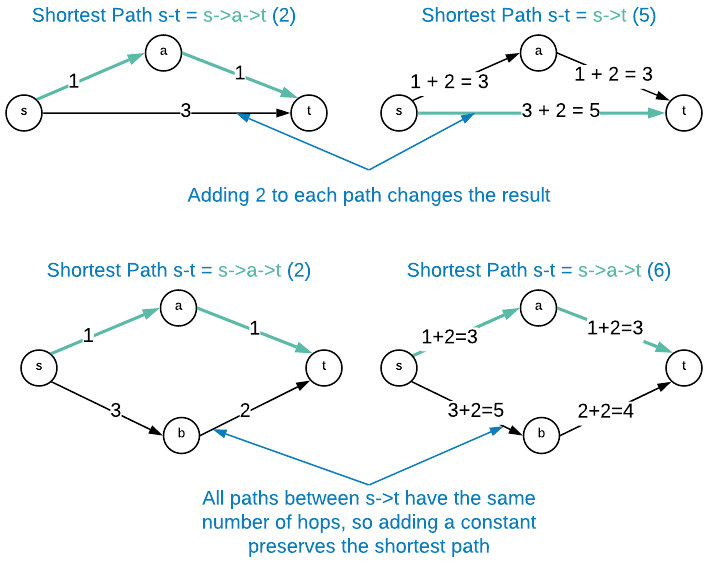# Johnson's Shortest Path

• Reduces the all pairs shortest path problem to $n$ iterations of Dijkstra’s algorithm while accommodating negative edge lengths.
• Reduces to:
• 1 invocation of Bellman-Ford
• $n$ invocations of Dijkstra’s

Suppose $G=(V,E)$ is a directed graph with edge lengths. Obtain $G^\prime$ from $G$ by adding a constant $M$ to every edge length. The shortest path between a source $s$ and a destination $t$ is guaranteed to be the same in $G$ and $G^\prime$ when all $s-t$ paths of $G$ have the same number of edges.Steps:

1. Add a new vertex ($S$) to graph with a zero weight edge to every existing vertex. This ensure that every vertex is reachable from a source vertex.
2. Compute the shortest path from $S$ to every other vertex using the Bellman-Ford algorithm.
• If a negative cycle exists, report and quit
3. Re-weight the vertices using $e_w = e_w + P_u - P_v$
• $e_w$ = edge weight
• $P_u$ = shortest path calculation for tail
• $P_v$ = shortest path calculation for head
4. Run Dijkstra’s algorithm for every $v \in V$
5. Restore the original weights by using $e_w = e_w - P_u + P_v$

## Applications

• List of commonly used application

## Asymptotic Complexity

$O(nm\log_2{n})$

## Pseudo Code

inputs:
input 1
input 2

side effect(s):
effect 1
effect 2

returns:
some value

invariants:
1 = 1

// intialize variables

function:
code here

code goes in here


Full Repo

Relevant Files: